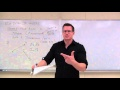Course

Calculus 3 by Leonard

4.77

(13)

Certificate

This course is free today and always will be, from start to finish you will pay nothing.Teacher

Leonard

This Channel is dedicated to quality mathematics education. It is absolutely FREE so Enjoy! Videos are organized in playlists and are course specific.

Course content preview

2h37m

1h10m

2h29m

2h11m

3h21m

2h32m

1h40m

2h04m

2h42m

2h12m

2h37m

1h02m

1h49m

2h14m

2h28m

1h51m

2h11m

2h37m

1h41m

Calculus 3 Lecture 13.8: Finding Extrema of Functions of 2 Variables (Max and Min)

3h38mWatch the 1st video of the course

Do not worry, it is free!

Course content2h37m

Calculus 3 Lecture 11.1: An Introduction to Vectors

Calculus 3 Lecture 11.1: An Introduction to Vectors: Discovering Vectors with focus on adding, subtracting, position vectors, unit vectors and magnitude.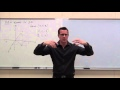1h10m

Calculus 3 Lecture 11.2: Vectors in 3-D Coordinate System

Calculus 3 Lecture 11.2: Vectors in 3-D Coordinate System: A study of point relationships and vectors in 3-D. Emphasis on position vectors, magnitude, and unit vectors.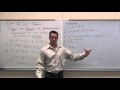2h29m

Calculus 3 Lecture 11.3: Using the Dot Product

Calculus 3 Lecture 11.3: Using the Dot Product: Explanation of the Dot Product, Finding the angle between two vectors including how the Dot Production show orthogonal vectors, Vector Projection, Work, and Direction angles.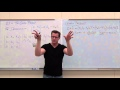2h11m

Calculus 3 Lecture 11.4: The Cross Product

Calculus 3 Lecture 11.4: The Cross Product: Finding the Cross Product of two vectors with Determinants, Using the Cross Product to find Mutually Orthogonal Vectors (with proofs), Torque, Area of a Parallelogram, Volume of a Parallelepiped, and Coplanar Vectors.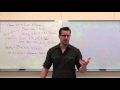3h21m

Calculus 3 Lecture 11.5: Lines and Planes in 3-D

Calculus 3 Lecture 11.5: Lines and Planes in 3-D: Parameter and Symmetric Equations of Lines, Intersection of Lines, Equations of Planes, Normals, Relationships between Lines and Planes, and Distances between 3-D objects.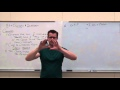2h32m

Calculus 3 Lecture 11.6: Cylinders and Surfaces in 3-D

Calculus 3 Lecture 11.6: Cylinders and Surfaces in 3-D: How to Identify and Sketch Cylinders and Surfaces. Shows step by step instruction for any cylinder, ellipsoid, hyperboloid, cone, paraboloid, and hyperbolic paraboloid. Focus is on HAND SKETCHING techniques.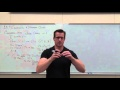1h40m

Calculus 3 Lecture 11.7: Using Cylindrical and Spherical Coordinates

Calculus 3 Lecture 11.7: Using Cylindrical and Spherical Coordinates: Show how to convert between Rectangular, Cylindrical, and Spherical coordinates AND how to convert between Rectangular, Cylindrical, and Spherical Equations.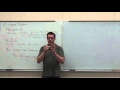2h04m

Calculus 3 Lecture 12.1: An Introduction To Vector Functions

Calculus 3 Lecture 12.1: An Introduction To Vector Functions: The interpretation of Vector Functions and How to graph Vector Functions. Focus is on the graphing of 3-D Vector Functions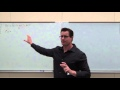2h42m

Calculus 3 Lecture 12.2: Derivatives and Integrals of Vector Functions

Calculus 3 Lecture 12.2: Derivatives and Integrals of Vector Functions: How to take Derivatives and Integrals of Vector Functions. Focus will be on the Tangent vector to space curves, finding tangent lines to vector functions, and Initial Value problems involving integrals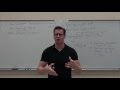2h12m

Calculus 3 Lecture 12.3: Arc Length/Parameterization, TNB (Frenet-Serret) Intro

Calculus 3 Lecture 12.3: Arc Length/Parameterization, TNB (Frenet-Serret) Intro: How to find the arc length and arc length function of a vector function (space curve). How to reparameterize a vector function by arc length. An introduction to Movable Frames; TNB Frames or Frenet-Serret Frames and what they mean.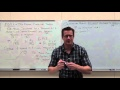2h37m

Calculus 3 Lecture 12.3/12.5: TNB (Frenet-Serret) Frames, Curvature, Torsion, Encapsulation

Calculus 3 Lecture 12.3/12.5: TNB (Frenet-Serret) Frames, Curvature, and Torsion: Focus on how to compute the TNB Frames as simply as possible and use the results to find the Curvature, Osculating circle, osculating plane, normal plane, and radius of curvature. Formulas are also given including how to perform vector encapsulation for 2-D functions.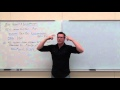1h02m

Calculus 3 Lecture 12.4: Velocity and Acceleration of Vector Functions

Calculus 3 Lecture 12.4: Velocity and Acceleration of Vector Functions: How to find the Velocity and Acceleration of a particle when the particular motion is described by a vector function (space curve). How to integrate to find a position function from acceleration or velocity. How to define acceleration as "T" and "N". A look at Projectile Motion.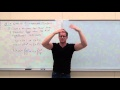1h49m

Calculus 3 Lecture 13.1: Intro to Multivariable Functions (Domain, Sketching, Level Curves)

Calculus 3 Lecture 13.1: Intro to Multivariable Functions (Domain, Sketching, Level Curves): Working with Multivariable Functions with an emphasis on finding Domain, Sketching Domain, Sketching Functions, and finding both Level Curves and Contour Plots.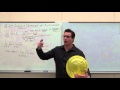2h14m

Calculus 3 Lecture 13.2: Limits and Continuity of Multivariable Functions (with Squeeze Th.)

Calculus 3 Lecture 13.2: Limits and Continuity of Multivariable Functions: How to show a limit exits or Does Not Exist for Multivariable Functions (including Squeeze Theorem). Also, how to determine regions of continuity.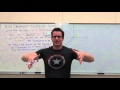2h28m

Calculus 3 Lecture 13.3: Partial Derivatives (Derivatives of Multivariable Functions)

Calculus 3 Lecture 13.3: Partial Derivatives (Derivatives of Multivariable Functions): How to find the slope of a tangent line to a surface in the X-Direction and Y-Direction. Focus is on the concept of a partial derivative and several examples. Also included is implicit differentiation and higher order partial derivatives.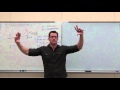1h51m

Calculus 3 Lecture 13.4: Finding Differentials of Multivariable Functions

Calculus 3 Lecture 13.4: Finding Differentials of Multivariable Functions: A review of Differentials from Calculus 1 and an extrapolation towards Differentials with more than 1 Independent Variable. Focus will be on the derivation of the idea of Differentials and the application of Differentials in Word Problems.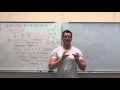2h11m

Calculus 3 Lecture 13.5: The Chain Rule for Multivariable Functions

Calculus 3 Lecture 13.5: The Chain Rule for Multivariable Functions: How to find derivatives of Multivariable Functions involving Parametrics and/or Compositions. Focus will be on deriving the Chain Rule and practice of examples.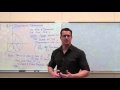2h37m

Calculus 3 Lecture 13.6: Finding Directional Derivatives and Gradients

Calculus 3 Lecture 13.6: Finding Directional Derivatives and Gradients: How to find a Directional Derivative along the path of any given vector. Also, an introduction to the Gradient and some basics on how it is used.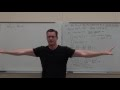1h41m

Calculus 3 Lecture 13.7: Finding Tangent Planes and Normal Lines to Surfaces

Calculus 3 Lecture 13.7: Finding Tangent Planes and Normal Lines to Surfaces: How to find a tangent plane and/or a normal line to any surface (multivariable function) at a point. This is based on the ability to find the gradient of a function.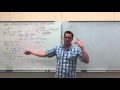3h38m

Calculus 3 Lecture 13.8: Finding Extrema of Functions of 2 Variables (Max and Min)

Calculus 3 Lecture 13.8: Finding Extrema of Functions of 2 Variables (Max and Min): How to find Relative (Local) and Absolute Extrema of 2 Variable Functions. Optimization is also discussed.